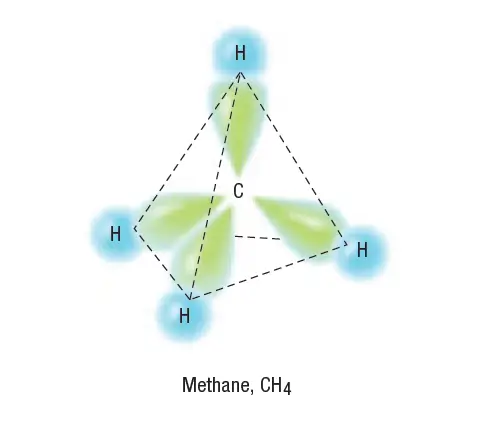# hybridization model & hybrid orbitals

In this post, we will explain the hybridization model & Hybrid orbitals in an atom.

## Hybridization model

To explain how the orbitals become rearranged when an atom forms covalent bonds, a special model is used. This model is called hybridization. The hybridization model is the mixing of two or more atomic orbitals of similar energies on the same atom to produce new hybrid atomic orbitals of equal energies.

Methane, CH4, provides a good example of how hybridization is used to explain the geometry of molecular orbitals. The orbital notation for a carbon atom shows that it has four valence electrons, two in the 2s orbital and two in the 2p orbitals.

## Hybridization of Carbon

The sp3 hybridization of carbon’s outer orbitals combines one s and three p orbitals to form four sp3 hybrid orbitals. Whenever hybridization occurs, the resulting hybrid orbitals are at an energy level between the levels of the orbitals that have combined.

## How does carbon form four equivalent, tetrahedrally arranged covalent bonds by orbital overlap with four other atoms?

We know from experiments that a methane molecule has tetrahedral geometry.

Two of carbon’s valence electrons occupy the 2s orbital, and two occupy the 2p orbitals.

Note that the 2s orbital and the 2p orbitals have different shapes. To achieve four equivalent bonds, carbon’s 2s and three 2p orbitals hybridize to form four new, identical orbitals called sp3 orbitals. Superscript 3 indicates that three p orbitals were included in the hybridization. The sp3 orbitals all have the same energy, which is greater than that of the 2s orbital but less than that of the 2p orbitals, as shown in figure 2.

Hybrid orbitals are orbitals of equal energy produced by the combination of two or more orbitals on the same atom. The number of hybrid orbitals produced equals the number of orbitals that have combined.
Bonding with carbon sp3 orbitals is shown in Figure 3 for a molecule of methane.Hybrid Orbitals – Bonds formed by the overlap of the 1s orbitals of hydrogen atoms and the sp3 orbitals of carbon

## Hybrid orbitals – definition

Hybrid orbitals are orbitals of equal energy produced by the combination of two or more orbitals on the same atom. The number of hybrid orbitals produced equals the number of orbitals that have combined.